maths > construction-basics

Geometrical Instruments

what you'll learn...

overview

•  compass

•  protractor

•  divider

•  set-squares or set-triangles

ruler / scale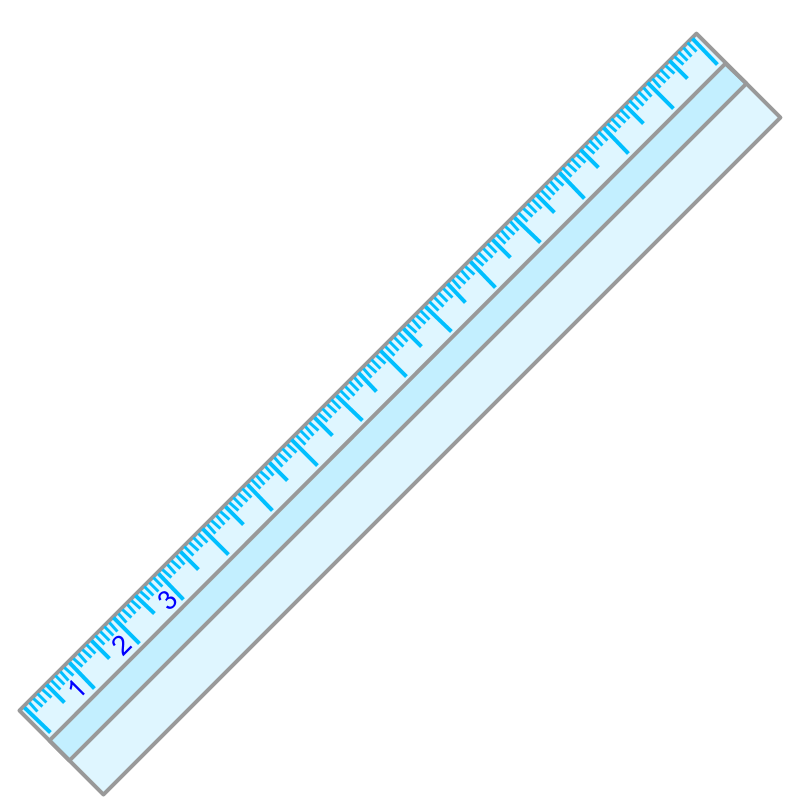The geometrical instrument in the figure is called a "ruler".

A "ruler" is used to construct a straight line.

The marks or graduations on the ruler show the length measure in millimeter and centimeter.

Other than to construct straight lines, A "ruler" is used to measure a line-segment.

The word "ruler" means: a straight stick.

The word "ruler" is derived from a root word meaning straight stick.

The phrase "graduated ruler" means: a straight stick with grade of length measure marked on them.

Note: A ruler is also referred with the name "scale".

summary

Graduated Ruler or scale : A ruler is a straight stick with grade of length measure marked. It is used to construct straight lines and measure a line-segment.

compass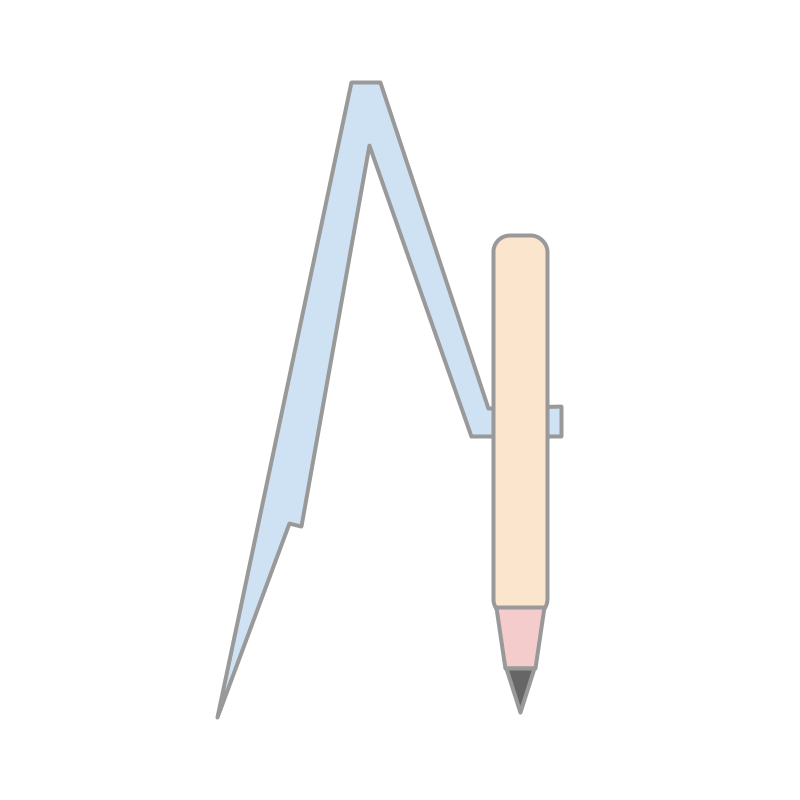The geometrical instrument in the figure is called a "compass".

A "compass" is used to construct an arc of given radius. The arc has points that are in equal distance from the center point.

The word "compass" is (possibly) from root words "com+pace" meaning "together+take-steps".

summary

Compass : An instrument to measure a length and construct an arc of a given radius.

divider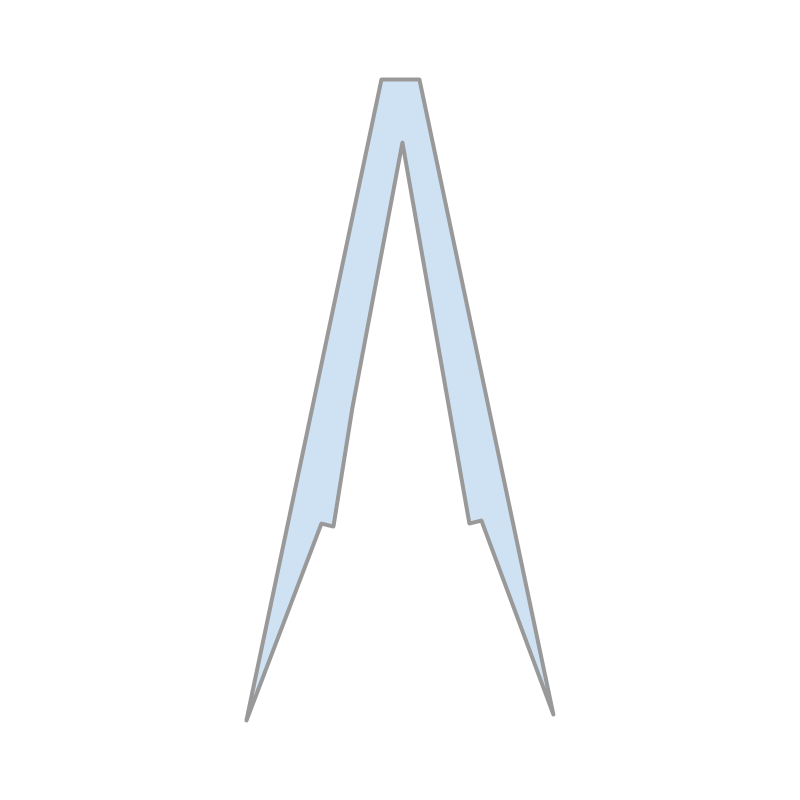The geometrical instrument in the figure is called a "divider".

A "divider" is used to measure the length between two points.

summary

Divider : is a geometrical instrument which is used to measure the length between two points.

protractor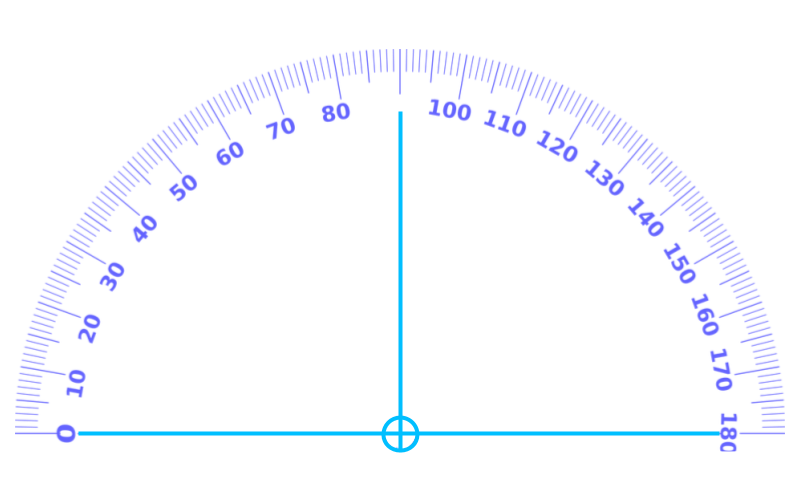The geometrical instrument in the figure is called a "protractor".

A "protractor" is used to mark an angle of given measure.

The word "Protractor" is from root words "protract+tor", which means "draw-out or lengthen a line".

summary

Protractor : is a geometrical instrument which is used to mark a measure of an angle.

set sequares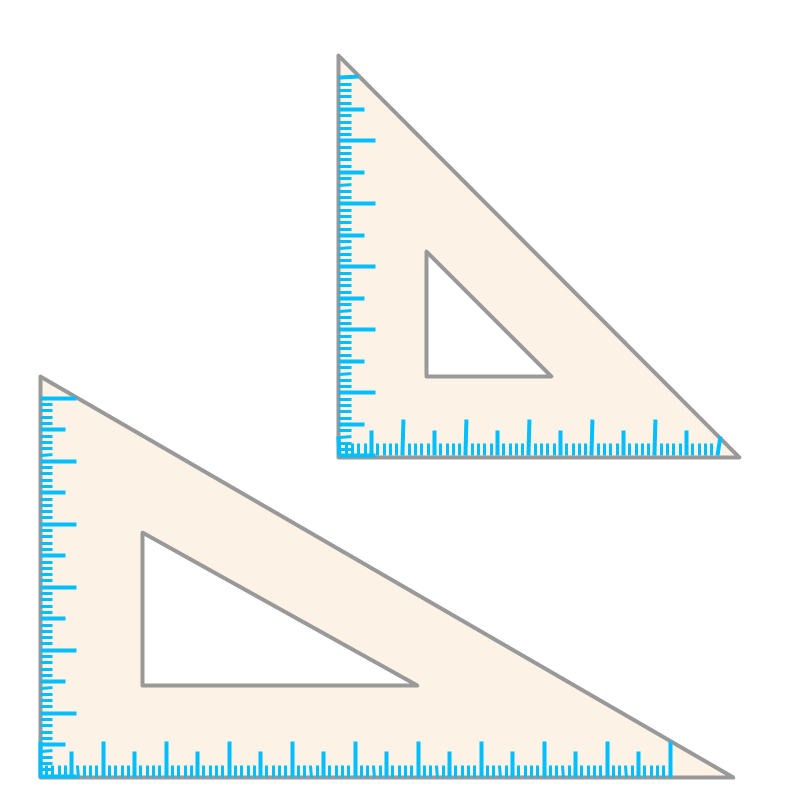The geometrical instrument in the figure is called a "set squares" or a "set triangles".

The name "set squares" calls by the two ${90}^{\circ }$${90}^{\circ}$ angles provided by the instruments.

The name "set triangles" calls by the shape of the two pieces. Both names are used.

A "set squares" is used

•  to construct perpendicular and parallel lines

•  to measure standard angles ${90}^{\circ }$${90}^{\circ}$, ${30}^{\circ }$${30}^{\circ}$, ${45}^{\circ }$${45}^{\circ}$, ${60}^{\circ }$${60}^{\circ}$ etc.

summary

Set Squares or Set Triangles : is a pair of triangular pieces used to construct parallel lines or to measure the standard angles ${90}^{\circ }$${90}^{\circ}$, ${30}^{\circ }$${30}^{\circ}$, ${45}^{\circ }$${45}^{\circ}$, ${60}^{\circ }$${60}^{\circ}$ etc.

summary

Graduated Ruler or scale : A ruler is a straight stick with grade of length measure marked. It is used to construct straight lines and measure a line-segment.

Compass : An instrument to measure a length and construct an arc of a given radius.

Divider : is a geometrical instrument which is used to measure the length between two points.

Protractor : is a geometrical instrument which is used to mark a measure of an angle.

Set Squares or Set Triangles : is a pair of triangular pieces used to construct parallel lines or to measure the standard angles ${90}^{\circ }$${90}^{\circ}$, ${30}^{\circ }$${30}^{\circ}$, ${45}^{\circ }$${45}^{\circ}$, ${60}^{\circ }$${60}^{\circ}$ etc.

Outline